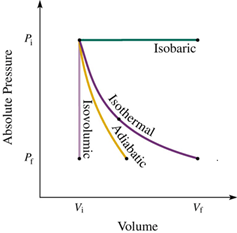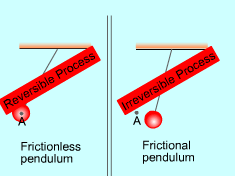×#### Thank you for registering.

One of our academic counsellors will contact you within 1 working day.

Click to Chat

1800-1023-196

+91-120-4616500

CART 0

• 0

MY CART (5)

Use Coupon: CART20 and get 20% off on all online Study Material

ITEM
DETAILS
MRP
DISCOUNT
FINAL PRICE
Total Price: Rs.

There are no items in this cart.
Continue Shopping• Complete Physics Course - Class 11
• OFFERED PRICE: Rs. 2,968
• View Details

```Reversible and Irreversible Process:-

Reversible Process:-It is a process which can be made to proceed in the reverse direction by a very slight change in its conditions so that the system passes through  the same states as in direct process, and at the conclusion of which the system and its surroundings acquire the initial conditions.

All isothermal and adiabatic process when allowed to proceed slowly, are reversible, provided there is no loss of energy against any type of resistance. Friction, viscosity etc. Examples of some reversible processes are given below.

Below are the locus of isothermal, isobaric, isochoric and adiabatic process.Examples of some reversible process:-(a) One gram of ice at 0°C requires 80 cal of heat to be converted into water at 0°C. Same amount of heat when taken out of water will convert it back to ice. So, fusion of ice is a reversible process.(b) In terms of the reaction involving hydrogenation and dehydrogenation of milled LiH, the process seems to be reversible.

(c) You can freeze orange juice to make an ice lolly and when you heat it the ice lolly become orange juice again. This is also an example of reversible process.

(d) One gram of water at 100°C gets converted into steam at 100°C by gaining 536 cal of heat. Same amount of heat when taken out of steam converts it back to water. So, vaporization of water is also a reversible process.

(e) Passage of electric current through a thermo-couple creates some temperature difference between the two junctions. Temperatures of the two junctions get reversed on reversing the direction of electric current. So ‘Peltier effect’ is also a reversible process.

Following basic conditions have to be fulfilled for a process to be reversible:-(a) The change must take place at a very slow rate.

(b) There should be no loss of energy due to the conduction or convection or dissipation of energy against any resistive effect like friction, viscosity etc.

(c) No heat should be converted  into magnetic or electric energy.

(d) The system must always be in thermal and mechanical equilibrium with the surrounding.

Irreversible Process:-

A process which cannot be made to be retraced in opposite direction by reversing the controlling factor is called an irreversible process.

The conditions mentioned above for the realisation of a reversible process are difficult to be met with, practically, since in every process some loss of energy is going to take place for one reason or the other. So most of the processes we come across in our daily life are irreversible processes.

Examples of some irreversible process:-Baking of a cake is an iireversible process.

(i) Work done against friction.

(ii) Joule’s heating effect.

(iii) Diffusion of gases into another.

(iv) Magnetic hysteresis.The reversible process should occur in infinitesimally small time.

In the reversible process all of the initial and final state of the system should be in equilibrium with each other.

During the irreversible process the various states of the system on the path of change from initial state to final state are not in equilibrium with each other.

During the irreversible process the entropy of the system increases decisively and it cannot be reduced back to its initial value.

The phenomenon of a system undergoing irreversible process is called as irreversibility.

In the irreversible process the initial state of the system and surroundings cannot be restored from the final state.

Problem 1:

Tweleve grams of Nitrogen (N2) in a steel tank are heated from 25.0 to 125ºC. (a) How many moles of nitrogen are present? (b) How much heat is transferred to the nitrogen?

Solution:

Number of moles (n) is equal to given mass of molecule (m) divided by molecular weight (W) of the molecule,

n = m/M

The heat transferred (Q) in a constant volume process is equal to,

Q = nCvΔT

Here Cv is the molar heat capacity at constant volume, ΔT (ΔT =Tf –Ti) is the raising temperature and n is the number of moles.

Thus, Q = nCvΔT

= nCv(Tf –Ti)

To find out the number of moles n of nitrogen, substitute 12 g for given mass m and 28 g/mol for M (molecular weight of nitrogen) in the equation n = m/M,

n = m/M

= (12 g)/(28 g/mol)

= 0.429 mol

Thus 0.429 moles of nitrogen are present.

Since nitrogen (N2) is a diatomic gas, therefore Cv = 5/2 R.

To obtain the heat Q which is transferred to the nitrogen, substitute 0.429 mol for n, 5/2 R for Cv and 125° C for Tf and 25° C for Ti in the equation Q = nCv(Tf –Ti),

Q = nCv(Tf –Ti)

= (0.429 mol) (5/2 R) (125° C-25° C)

= (0.429 mol) (5/2 ×8.31 J/mol. K) ((125 + 273) K-(25 + 273) K)      (Since, R = 8.31 J/mol. K)

= (0.429 mol) (5/2 ×8.31 J/mol. K) (398 K-298 K)

= (0.429 mol) (5/2 ×8.31 J/mol. K) (100 K)

= 891 J

From the above observation we conclude that, the heat Q which is transferred to the nitrogen would be 891 J.

_______________________________________________________________________

Problem 2:

A thermometer of mass 0.055 kg and heat capacity 46.1 J/K reads 15.0ºC. It is then completely immersed in 0.300 kg of water and it comes to the same final temperature as the water. If the thermometer reads 44.4 ºC, what was the temperature of the water before insertion of the thermometer, neglecting other heat losses?

Solution:

In accordance to the law of conservation of energy, for a thermodynamic system, in which internal is the only type of energy the system may have, the law of conservation of energy  may be expressed as,

Q + W = ΔEint

Here Q is the energy transferred between the system and its environment, W is the work done on or by the system and ΔEint is the change in the internal energy of the system.

The heat capacity C of a body as the ratio of amount of heat energy Q transferred to a body in any process to its corresponding temperature change ΔT.

C = Q/ΔT

So, Q = C ΔT

The heat capacity per unit mass of a body, called specific heat capacity or usually just specific heat, is characteristic of the material of which the body is composed.

c = C/m

= Q/mΔT

So, Q = c mΔT

The heat transfers for the water Qw is,

Qw = mwcw (Tf –Ti)

Here, mass of water is mw, specific heat capacity of water is cw, final temperature is Tf and initial temperature is Ti.

The heat transfers for the thermometer Qt is

Qt = CtΔTt

Here, heat capacity of thermometer is Ct and ΔTt is the temperature difference.

As the internal energy of the system is zero and there is no work is done, therefore substitute ΔEint = 0 and W = 0 in the equation Q + W = ΔEint,

Q + W = ΔEint

Q + 0= 0

So, Q = 0

Or, Qw + Qt = 0

mwcw (Tf –Ti)+ CtΔTt = 0

So, Ti = (mwcw Tf + CtΔTt )/ mwcw

Here ΔTt = 44.4 ° C - 15.0 ° C

=  29.4 ° C

To obtain the temperature of the water before insertion Ti of the thermometer, substitute 0.3 kg for mw, 4190 J/kg.m for cw, 44.4 ° C for Tf, 46.1 J/K for Ct and 29.4 ° C for ΔTt in the equation Ti = (mwcw Tf + CtΔTt )/ mwcw,

Ti = (mwcw Tf + CtΔTt )/ mwcw

= [(0.3 kg) (4190 J/kg.m) (44.4 ° C) + (46.1 J/K) (29.4 ° C)] /[(0.3 kg) (4190 J/kg.m)]

=45.5 ° C

From the above observation we conclude that, the temperature of the water before insertion of the thermometer was 45.5 ° C.

_________________________________________________________________________________

Problem 3:

An aluminum electric kettle of mass 0.560 kg contains a 2.40 kW heating element. It is filled with 0.640 L of water at 12.0ºC. How long will it take (a) for boiling to begin and (b) for the kettle to boil dry? (Assume that the temperature of the kettle does not exceed 100ºC at any time.)

Solution:

The amount of heat per unit mass that must be transferred to produce a phase change is called the latent L for the process. The total heat transferred in a phase change is then

Q = Lm,

Here m is the mass of the sample that changes phase. The heat transferred during melting or freezing is called the heat of fusion.

The heat which is given to a body of mass m, whose material has a specific heat c, to increase its temperature from initial temperature Ti to final temperature Tf will be,

Q= mc (Tf - Ti)

= mcΔT

Mass m is equal to density ρ of object times volume V of the object.

m = ρV

Time t is equal to the heat energy Q divided by power P.

t = Q/P

(a) The time t taken by the electric kettle for boiling to begin will be,

t = Q/P

= [maca +ρwVwcw]ΔT/P

= [maca +ρwVwcw] (Tf - Ti)/P

Here, mass of aluminum electric kettle is ma, specific heat of aluminum is ca, density of water is ρw, volume of water is Vw, specific heat of aluminum is cw, final temperature is Tf , initial temperature is Ti and power is P.

To obtain the time t taken by the electric kettle for boiling to begin, substitute 0.56 kg for ma, 900 J/kg. K for ca, 998 kg/m3 for ρw, 0.640 L for Vw, 4190 J/kg.K for cw, 100°C for Tf and 12° C for Ti and 2.40 kW for P in the equation t =[maca +ρwVwcw] (Tf - Ti)/P,

t =[maca +ρwVwcw] (Tf - Ti)/P

= [(0.56 kg) (900 J/kg. K) + (998 kg/m3) (0.640 L) (4190 J/kg.K)] (100°C -12° C)/2.40 kW

= [(0.56 kg) (900 J/kg. K) + (998 kg/m3) (0.640 L×10-3 m3/1 L) (4190 J/kg.K)] ((100+273) K –(12+273) K)/(2.40 kW×103 W/1 kW)

= [(0.56 kg) (900 J/kg. K) + (998 kg/m3) (0.640×10-3m3) (4190 J/kg.K)] (373 K –285 K)/(2400 W)

= 117 s

From the above observation we conclude that, the time t taken by the electric kettle for boiling to begin would be 117 s.

(b) The time t for the kettle to boil dry will be,

t = Q/P

= ρwVwLw/P

Here density of water is ρw, volume of water is Vw, latent heat of water is Lw and power is P.

To obtain the time t for the kettle to boil dry, substitute 998 kg/m3 for ρw, 0.640 L for Vw, 2256×103 J/kg for Lw and 2.40 kW for P in the equation t = ρwVwLw/P,

t = ρwVwLw/P

= (998 kg/m3) (0.640 L) (2256×103 J/kg)/( 2.40 kW)

= (998 kg/m3) (0.640 L×10-3 m3/1 L) (2256×103 J/kg)/( 2.40 kW×103 W/1 kW)

= (998 kg/m3) (0.640 ×10-3 m3) (2256×103 J/kg)/( 2400 W)

= 600 s

From the above observation we conclude that, the time t for the kettle to boil dry would be 600 s.Question 1

Which of the following process is reversible?

(a) Transfer of heat by conduction

(b) Transfer of heat by radiation

(c) Isothermal compression

(d) Electrical heating of nichrome wire

Question 2

P-V diagram of an isochoric change is:

(a) a line parallel to P-axis.

(b) a line parallel to V-axis

(c) a line inclined at an angle 45º with V-axis

(d) a line inclined at 135º with V-axis.

Question 3

Which of these an irreversible change?

(a) Candle wax melting

(b) candle wax burning

(c) Ice cream melting

Question 1

Which of these a reversible change?

(a) Metal rusting

(b) Baking a cake

(c) Freezing orange juice to make an ice lolly

Question 4

Bursting of cracker is a/an

(a) reversible change

(b) irreversible change

(c) both reversible and irreversible

(d) None of theseQ.1
Q.2
Q.3
Q.4
Q.5

c

a

b

c

b

Related Resources:-

You might like to Specific Heat Capacity.

For getting an idea of the type of questions asked, refer the  Previous Year Question Papers.

To read more, Buy study materials of Thermodynamics comprising study notes, revision notes, video lectures, previous year solved questions etc. Also browse for more study materials on Chemistry here.
```### Course Features

• 101 Video Lectures
• Revision Notes
• Previous Year Papers
• Mind Map
• Study Planner
• NCERT Solutions
• Discussion Forum
• Test paper with Video Solution